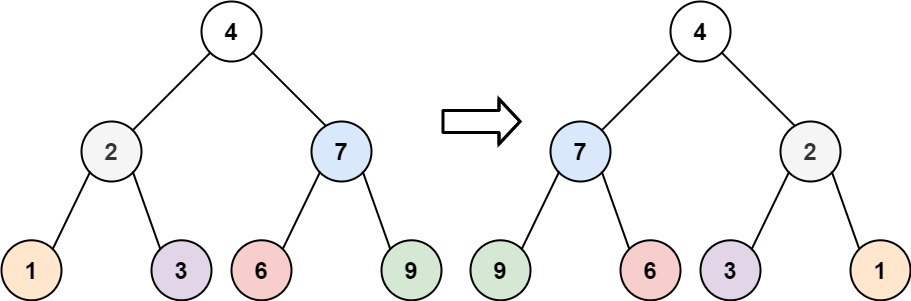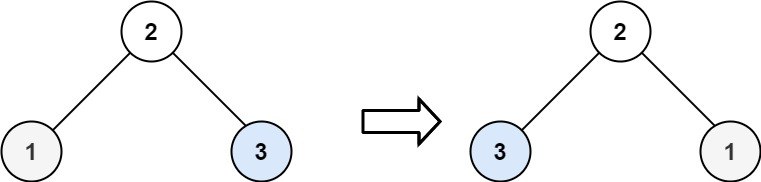## C# || How To Invert Binary Tree Using C#The following is a module with functions which demonstrates how to invert a binary tree using C#.

1. Invert Tree – Problem Statement

Given the root of a binary tree, invert the tree, and return its root.

Example 1:``` Input: root = [4,2,7,1,3,6,9] Output: [4,7,2,9,6,3,1] ```

Example 2:``` Input: root = [2,1,3] Output: [2,3,1] ```

Example 3:

``` Input: root = [] Output: [] ```

2. Invert Tree – Solution

The following is a solution which demonstrates how to invert a binary tree.

An inverted Binary Tree is simply a Binary Tree whose left and right children are swapped.

This solution:

• Traverses the left subtree
• Traverses the right subtree
• When both trees have been traversed, swap left and right child subtrees

``` 2. Invert Tree - Solution C# // ============================================================================ // Author: Kenneth Perkins // Date: Oct 26, 2021 // Taken From: http://programmingnotes.org/ // File: Solution.cs // Description: Demonstrates how to invert a binary tree // ============================================================================ /** * Definition for a binary tree node. * public class TreeNode { * public int val; * public TreeNode left; * public TreeNode right; * public TreeNode(int val=0, TreeNode left=null, TreeNode right=null) { * this.val = val; * this.left = left; * this.right = right; * } * } */ public class Solution { public TreeNode InvertTree(TreeNode root) { Traverse(root); return root; } private void Traverse(TreeNode node) { if (node == null) { return; } // Keep traversing left and right nodes Traverse(node.left); Traverse(node.right); // Root has been visited, swap left and right child nodes var temp = node.left; node.left = node.right; node.right = temp; } }// http://programmingnotes.org/ 1234567891011121314151617181920212223242526272829303132333435363738394041 // ============================================================================//    Author: Kenneth Perkins//    Date:   Oct 26, 2021//    Taken From: http://programmingnotes.org///    File:  Solution.cs//    Description: Demonstrates how to invert a binary tree// ============================================================================/** * Definition for a binary tree node. * public class TreeNode { *     public int val; *     public TreeNode left; *     public TreeNode right; *     public TreeNode(int val=0, TreeNode left=null, TreeNode right=null) { *         this.val = val; *         this.left = left; *         this.right = right; *     } * } */public class Solution {    public TreeNode InvertTree(TreeNode root) {        Traverse(root);        return root;    }     private void Traverse(TreeNode node) {        if (node == null) {            return;        }         // Keep traversing left and right nodes        Traverse(node.left);        Traverse(node.right);         // Root has been visited, swap left and right child nodes        var temp = node.left;        node.left = node.right;        node.right = temp;    }}// http://programmingnotes.org/ ```

QUICK NOTES:
The highlighted lines are sections of interest to look out for.

The code is heavily commented, so no further insight is necessary. If you have any questions, feel free to leave a comment below.

Once compiled, you should get this as your output for the example cases:

``` [4,7,2,9,6,3,1] [2,3,1] [] ```